# RRB Clerk Prelims Reasoning Ability Questions 2021 (Day-05)

Dear Aspirants, Our IBPS Guide team is providing new series of Reasoning Questions for RRB Clerk Prelims 2021 so the aspirants can practice it on a daily basis. These questions are framed by our skilled experts after understanding your needs thoroughly. Aspirants can practice these new series questions daily to familiarize with the exact exam pattern and make your preparation effective.

Start Quiz

Syllogism

Direction (1-5): Read both the conclusions and then decide which of the given conclusions logically follows from the given statements disregarding commonly known facts.

1) Statements:

Only a few stars are planets

No galaxy is a planet

Conclusions:

I)Some stars are not galaxies

II)No galaxy is star

A.only conclusion I follows

B.only conclusion II follows

C.either conclusion I or II follows

D.Both conclusion I and II follows

E.none follows

2) Statements:

Only a few schools are colleges

No student is school

All schools are parks

Conclusions:

I)All schools are colleges

II)Some colleges are not students

A.Only conclusion I follows

B.Only conclusion II follows

C.Both conclusions I and II follows

D.Either conclusions I or conclusions II follows

E.Neither conclusions I nor conclusions II follows

3) Statements:

All books are tables

Only a few pens are books

Conclusions:

I)All tables can be pens.

II)All pens can be books.

A.Only conclusion I follows

B.Only conclusion II follows

C.Both conclusions I and II follows

D.Either conclusions I or conclusions II follows

E.None follows

4) Statements:

No copper is aluminium

Only a few copper is silver

Conclusions:

I)All aluminium is silvers

II)No copper is silver

A.Only conclusion I follows

B.Only conclusion II follows

C.Both conclusions I and II follow

D.Either conclusions I or conclusions II follows

E.None follows

5) Statements:

Only a few diagrams are circle.

All squares are circles

Conclusion:

I)All diagrams are squares are a possibility.

II)Some square can be Diagrams.

A.Only conclusion I follows

B.Only conclusion II follows

C.Both conclusions I and II follows

D.Either conclusions I or conclusions II follows

E.None follows

Coding decoding

Direction (6-8): Study the following information carefully and answer the questions below.

In a certain code language,

“Rate image data task’’ is coded as “jh km yu od’’

“Burn security rainfall’’ is coded as “ds po na’’

“Rainbow success task’’ is coded as “bu ra yu’’

“Security data burn success’’ is coded as “ds po km bu’’

6) What is the code for ‘image’?

A.jh

B.od

C.km

D.either a or b

E.either b or c

7) What does code ‘bu’ stands for?

A.rainbow

B.success

D.security

E.burn

8) Which of the following code may be for “grow rate rainfall’’?

A.jh sh na

B.km na od

C.bu ra jh

D.od km yu

E.none of these

#### Miscellaneous

9) If it is possible to make a meaningful word with the letter ‘K’, ‘O’, ‘E’, ‘Y’, and ‘L’ using each letter only once, what will be the last letter of that word? If more than one such word can be formed, mark your answer as ‘X’ if no such word can be formed, your answer will be ‘y’?

A.L

B.O

C.E

D.x

E.y

10) If all the digits of the number ‘78631524’ are arranged in descending order from left to right, then what is the difference between the first and sixth digit of the number from the left end?

A.6

B.7

C.5

D.2

E.1

The least possible diagram is as follows: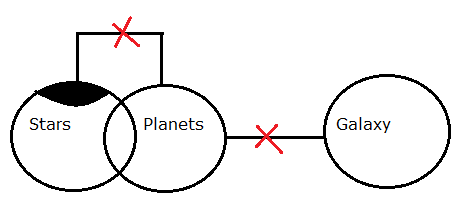The least possible diagram is as follows: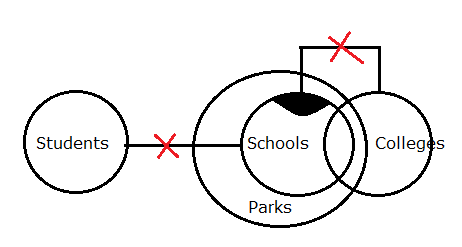The least possible diagram is as follows: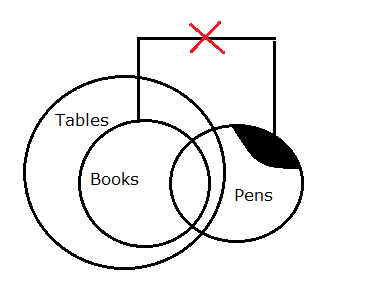The least possible diagram is as follows: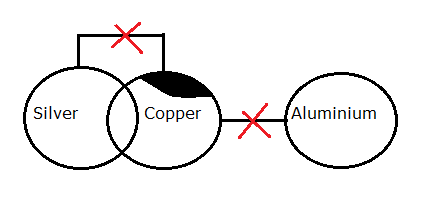The least possible diagram is as follows: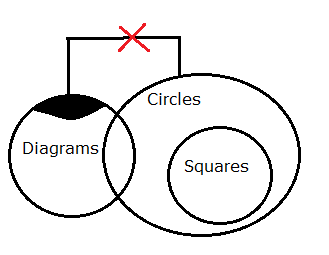Directions (6-8) :

The final table is shown below: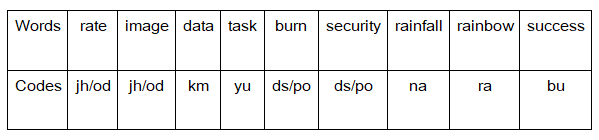The given letters are ‘K’, ‘O’, ‘E’, ‘Y’, and ‘L’

The meaningful word that can be formed after arranging these letters is ‘YOKEL’ which means gullible countryman in a small area

The last letter of the word is ‘L’